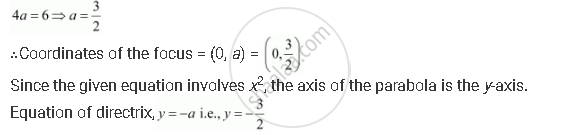CBSE (Arts) Class 11CBSE
Share
Notifications

View all notifications
Books Shortlist
Your shortlist is empty

# Find the Coordinates of the Focus, Axis of the Parabola, the Equation of Directrix and the Length of the Latus Rectum for X2 = 6y - CBSE (Arts) Class 11 - Mathematics

Login
Create free account

Forgot password?
ConceptParabola Standard Equations of Parabola

#### Question

Find the coordinates of the focus, axis of the parabola, the equation of directrix and the length of the latus rectum for x2 = 6y

#### Solution

The given equation is x2 = 6y.

Here, the coefficient of is positive. Hence, the parabola opens upwards.

On comparing this equation with x2 = 4ay, we obtainLength of latus rectum = 4a = 6

Is there an error in this question or solution?

#### APPEARS IN

Solution Find the Coordinates of the Focus, Axis of the Parabola, the Equation of Directrix and the Length of the Latus Rectum for X2 = 6y Concept: Parabola - Standard Equations of Parabola.
S